﻿

### Counting the generalized twisted fields

#### Abstract

In this paper we exploit a theorem of Biliotti, Jha, and Johnson exhibiting a procedure to count the number of non- isotopic generalized twisted fields of orders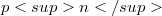$pn$ where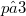$p ≥ 3$ which is denoted by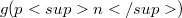$g(pn)$.We show that$g (pn)$ is a polynomial in p that is sharply bounded below by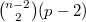${n-2 \choose 2}(p-2)$ and bounded above by a polynomial of degree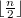$\lfloor{n \over 2}\rfloor$.

DOI Code: 10.1285/i15900932v27n1p53

Keywords: Semifield; Generalized twisted field; Projective plane; Finite geometry

Full Text: PDF PS